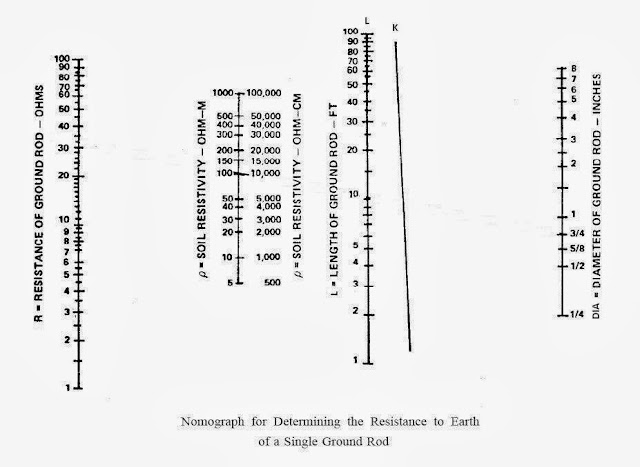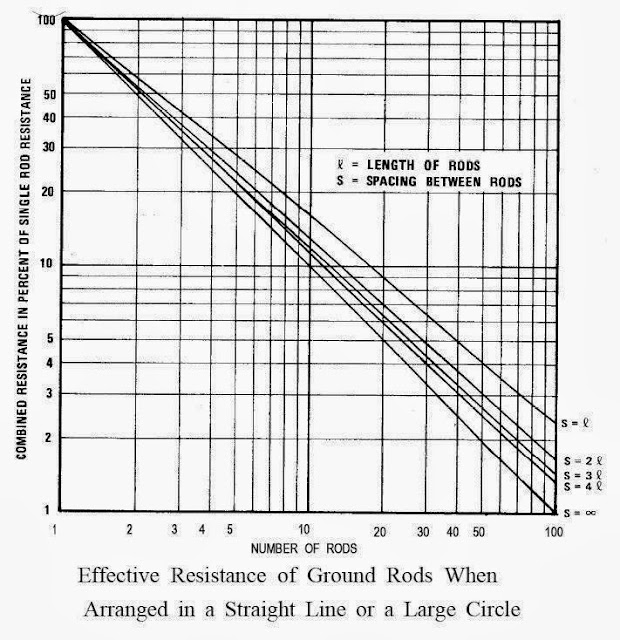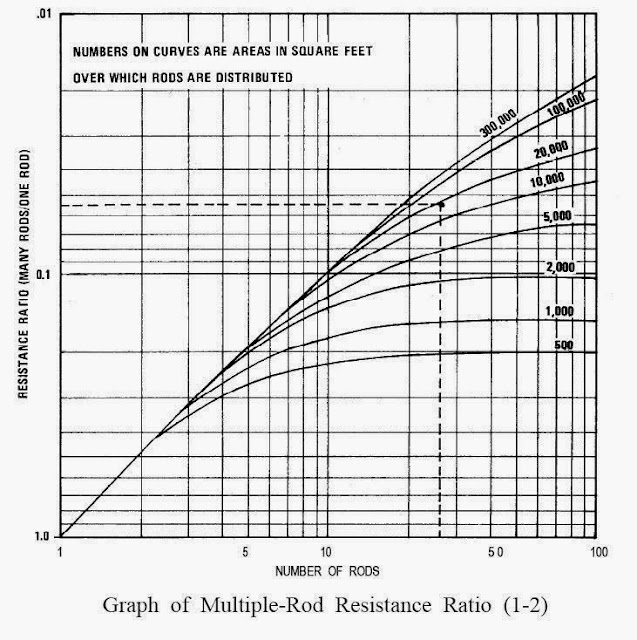### Grounding Design Calculations – Part Three

I indicated that the Earthing Systems Design Steps process has (3) main steps:

 Earthing Systems Design Steps A grounding system design process has (3) main steps: Data Collection, Data Analysis, Grounding Design Calculations.

And I explained the first step: Data Collection in the following Articles:

I explained the second step: Data Analysis in the following Articles:

And I explained What we are going to design for grounding system in any building in the following Articles:

And, in Article
Grounding Design Calculations – Part One ", I indicated the following:

 Grounding System Design Calculations according to type of the building The procedures for performing the Grounding System Design Calculations can differ slightly according to the type of the building as follows: Domestic, commercial and industrial premises, High and medium voltage electricity substations.

 First: Domestic, commercial and industrial premises We mean by domestic, commercial and industrial premises, all installations up to 1,000 V ac and 1,500 V dc - between phases, with some minor exceptions.

 Methods of Grounding Design Calculations There are many methods can be used for performing Grounding System Design Calculations But the common methods are: Equations Method, Nomographs Method, Excel Spreadsheets Method, Tables Method, Online Earthing Calculators Method, Software Programs Method.

In this Article, I explained the first method of grounding design calculations: Equations Method.

Also, in Article " Grounding Design Calculations – Part Two ", I explain Some solved examples for grounding design calculations using the Equations Method.

Today, I will explain the Other Methods for Performing of Grounding System Design Calculations.

 Second: Nomographs Method

 Definition: A Nomograph is a mathematical tool consisting of several nonlinear scales (see fig.1) on which known values can be plotted and the desired unknown value can be derived by simply connecting the points with a straightedge and finding the resultant by reading the intersecting point on the desired scale.

 Factors That Affect Earth Resistance Of The Electrode The resistance of a rod Rr in ohms (Ω) may be calculated from: Where: ρ is the resistivity of soil, in ohm metres (Ωm); L is the length of the electrode, in metres (m); d is the diameter of the rod, in metres (m). From the above equation, the factors that affect earth resistance of the electrode are: Earth resistivity: Changing the resistivity can be done by chemical treatment of the soil. Diameter of rod: Change of diameter has little effect on the overall value of resistance. Length of rod: The simplest and most effective way to change the overall value of resistance is changing the Length (Depth) of the driven rod.

 Uses of Nomographs The Nomographs can be a helpful guide in meeting the established standard for a minimum earth resistance as follows: If you have a given earth-electrode system and find that your Megger instrument reading is too high, the graph can be used to show what you must do to lower the value by changing the three variable conditions that affect earth resistance of the electrode which are: Earth resistivity, Length of rod, Diameter of rod. The Nomograph using is severely limited in several aspects as follows: The range of soil resistivity is effectively limited to around 11,000 ohms-cm. Soils are higher in resistivity than 11,000 ohms-cm nearly everywhere. It can only provide the length/diameter of a single grounding electrode. This is inadequate to meet the ground resistance requirement for sensitive equipment. The Nomographs is applied only when the earth electrode is rod electrode type.

 Application Of Nomographs Method The Nomographs method is applied for two cases of rod electrodes as follows: Case#1: for a single earth rod. Case#2: for multiple Rod electrode system.

 Case#1: For A Single Earth Rod For a single earth rod, two types of Nomographs are existing: First: Nomograph to calculate resistance of a single earth rod directly from the Wenner 4-electrode method readings, Second: Nomograph relating the basic factors affecting the earth resistance of a single earth rod.

First:  Nomograph to calculate resistance of a single earth rod directly from the Wenner 4-electrode method readings

This Nomograph is another method of calculating the ground resistance of an earth electrode when the electrode dimensions and the earth resistivity are known.

The resistance of an electrode may be calculated directly from the readings obtained from the Wenner 4-electrode method of soil resistivity test, if the test electrodes are spaced by a distance equal to the length of the electrode to be used.

The resistance is then given by the formula

Where:

L is the length of the intended electrode or the spacing between the test electrodes im meters
d is the diameter of the intended electrode in mm
R is the resistance of the intended electrode in ohms

1.1 Nomograph structure: (see fig.1)Fig.1

The Nomographs in this case will consist of (5) scales:

• Scale # (1); L scale: it represents the earth electrode length in (meter) or represents the distance between test stakes for Wenner method which used to measure the earth resistance,
• Scale # (2); d Scale: it represents the earth electrode diameter in (mm),
• Scale # (3); Turning ordinate scale,
• Scale # (4); r Scale: it represents Wenner 4-point test reading in (ohms),
• Scale # (5); R Scale: it represents the required resistance of the earth electrode in (ohms).

Note:

Scales # (4) and # (5) may be multiplied by a common factor to use for higher values not indicated in original scales.

1.2 Prerequisites:

Knowing of the following data:

• The Rod electrode dimensions (Length L in meter and Diammeter d in mm),
• Wenner 4-point test reading in (ohms).

1.3 Method Steps:

• Select given Rod length on Scale # (1); L scale
• Select given Rod diameter on Scale # (2); d Scale
• Place straightedge from L scale point to d scale point, and allow intersecting with Scale # (3); Turning ordinate scale to get point (A)
• Select given Wenner 4-point reading on Scale # (4); r Scale
• Place straightedge from point (A) on Scale # (3); Turning ordinate scale to point on Scale # (4); r Scale, and allow intersecting with Scale # (5); R Scale
• Read the value of intersection point on Scale # (5); R Scale, it represents the resistance of the earth electrode in (ohms).

Example#1:

Find a resistance of 10 mm diameter stakes, 6.7 m long, if the reading from the Wenner 4 point test is 1 ohm.

Solution:

• Place straightedge from 6.7 on scale #1 to cut scale #2 at 10, extend the straightedge to intersect with turning ordinate scale,
• Mark turning ordinate from where a straight edge trough 1 on scale #4 to cut scale #5,
• Read the value of intersection point on Scale # (5); R Scale, it will be 7.6 ohms.

Second:  Nomograph relating the basic factors affecting the earth resistance of a single earth rod

2.1 Nomograph Structure: (see fig.2)

The Nomographs in this case will consist of the (5) scales:Fig.2

• R Scale: it represents the resistance of the earth electrode in (ohms),
• ρ Scale: it represents the earth resistivity in (ohm-cm),
• L scale: it represents the earth electrode length in (feet),
• K Scale: turning ordinate scale,
• DIA Scale: it represents the earth electrode diameter in (inches).

2.2 Methods Steps:

We have two methods for applying the Nomograph relating the basic factors affecting the earth resistance of a single earth rod as follows:

A- finding the depth needed to achieve the desired resistance,
B- Finding the resistance of a single earth rod

2.2.A Finding The Depth Needed To Achieve The Desired Resistance

2.2.A.1  Prerequisites:

Knowing of the following data:

• The Rod electrode Diammeter DIA in inches,
• The earth resistivity ρ in ohm-cm,
• The desired system ground resistance in ohm.

2.2.A.2 Method Steps:

• Select desired resistance on R Scale
• Select apparent resistivity on ρ Scale.
• Lay straightedge from point on R scale to point on ρ Scale, and allow intersecting with K scale.
• Mark K scale point.
• Select given diameter on DIA Scale.
• Lay straightedge from point on K scale to point on DIA scale, and allow intersecting with L scale.
• Point on L scale will be the rod depth required to achieve desired resistance on R scale.

Example#2:

Find the rod depth needed to achieve a desired resistance of 10 ohms, if the soil resistivity 6515 ohm-centimeters and the rod diameter is 5/8 inches.

Solution:

• Put a dot at 10 ohms on the R scale as it is our desired resistance.
• Put a dot at 6515 on the ρ scale representing our soil resistivity measurement (do your best to approximate the location of this point between the 5000 and 10000 hash marks).
• Make a straightedge and draw a line between the dots we placed on the R and ρ scales and let the line intersect with the K scale and place a dot on the intersecting point.
• Put a dot at 5/8 hash mark on the DIA scale representing our rod diameter.
• make a straightedge and draw a line from the 5/8 hash mark on the DIA scale representing our rod diameter through the dot on the K scale and continue through to intersect with the L scale and place a dot on the L scale at this intersecting point.
• Read the value at this intersecting point on L scale, it will be 30 feet.

Note to Example#2:

• In many cases, this is not practical to drive deep rods. The alternative is to drive two or more rods to get the desired results, so a monograph of multiple Rod electrode system will be needed in this case and this is will be explained in below.

2.2.B Finding The Resistance Of A Single Earth Rod

2.2.B.1  Prerequisites:

Knowing of the following data:

• The Rod electrode Diammeter DIA in inches, and rod depth in feet,
• The earth resistivity ρ in ohm-cm.

2.2.B.2 Method Steps:

• Select given Rod diameter on DIA scale.
• Select given Rod length(depth) on L scale.
• Place straightedge from DIA scale point to L scale point, and allow intersecting with K Scale: turning ordinate scale to get point (A).
• Select given earth resistivity on ρ Scale.
• Place straightedge from point (A) on K Scale; Turning ordinate scale to point on ρ Scale, and allow intersecting with R Scale.
• Read the value of intersection point on R Scale, it represents the resistance of the earth electrode in (ohms).

Example#3:

Find a resistance of 5/8 inch rod diameter, 10 feet long, if earth resistivity is 4020 ohm-cm.

Solution:

Example#4:

If using a larger-diameter electrode at a deeper depth with respect to example#3, what will be grounding electrode resistance improvement? Noting that the new rod diameter is 3/4 inch and the new depth will be 20 feet. (Same earth resistivity)

Solution:

Example#5:

A 5/8-in. rod driven 10 ft into the soil and The Megger instrument indicates an earth resistance of 6.6 ohm but the desired resistance is 4 ohm. Find the required depth to give a 4 ohm earth resistance.

Solution:

Step#1: determine the earth resistivity
• With a ruler, draw a line from the 10-ft point in the L scale to the 5/8-inche point in the DIA scale; this gives a reference point where the line crosses the K scale.
• Connect this reference point with 6.6 ohm, the measured resistance on the R scale.
• Read the value of earth resistivity when this line crosses the ρ scale. The value is 2000 ohm-cm.

Step#2: determine the required rod depth for a 4 ohm earth resistance

• Draw a line from 4 ohm point on the R scale through the 2000 point on the p scale until you cross the K scale. The dashed line shows this step.
• Now, assuming rod diameter unchanged, connect the 5/8 point on DIA scale through your new reference point on K scale and extend the line to L scale. This gives the required rod depth for the 4 ohm resistance value which is 20 feet.

Notes for Example#5:

• In the new depth, a new instrument reading to check the value, because earth resistivity may not be constant as the nomograph assumes.
• Another way to reduce the earth resistance, in example#5 above, would be to lower the earth resistivity. if you draw a line from a reference point 1 (leaving rod depth and diameter unchanged), you would need to reduce earth resistivity to about 1000 ohm-cm to give the required 4 ohm earth resistance. You could do this by chemical treatment, but normally the deeper rod is the easier way.

Case#2: For Multiple Rod Electrode System

Monograph for Multiple Rod Electrode System is a means of calculating the resistance of an electrode system in respect of a number of electrodes driven in line, around the perimeter of a square site or forming a solid square when the electrodes are spaced their length apart ( space between each two electrodes = length of one electrode).

1.1 Nomograph Structure: (see fig.3)Fig.3

This monograph consists of (5) scales as follows:

• Scale# (1): calculated or measured resistance of one electrode in ohms,
• Scale# (2): Total earth resistance of electrode system,
• Scale# (3): number of electrodes driven in Line.
• Scale# (4): number of equally spaced electrodes driven to form a hollow square pattern.
• Scale# (5): number of equally spaced electrodes driven to form a solid square pattern.

1.2 Prerequisites:

Knowing of the following data:

• Calculated or measured resistance of one electrode in ohms,
• Electrode system shape,
• Number of electrodes.

1.3 Method Steps:

• Select calculated/measured earth resistance of one electrode on scale# (1).
• Select the given electrode arrangement on scale# (3).
• Draw straightedge between the known value of a single electrode on scale# (1) and the electrode arrangement on scale# (3).
• Read the value of the intersection point on scale# (2), it will be the resistance of the parallel connected electrodes to earth.

Note:

• Although the nomograph is in respect of square sites, it may be used for rectangular sites having the same number of electrodes if the narrower site dimension is greater than (3) electrode lengths.

Example#6:

For two 5 meter rods, each 10 ohm individual resistance, spaced 5 meter apart, what is the combined earth resistance?

Solution:

• Select 10 ohm on scale# (1)
• Select the line electrode arrangement for two electrodes on scale# (3)
• Draw straightedge between the two points
• Read the value of the intersection point on scale# (2), it equal 5.4 ohm
• So, the combined earth resistance = 5.4 ohm

Example#7:

an earthing electrode system of A holow square configuration consisting of 20 rod electodes ( each side has 6 rods) what is the combined earth resistance of these electrodes knowing that the soil resistivity is 10,000 ohm-cm, Rod electrode depth is 10 feet and rod diameter is 3/4 inch.

Solution:

Step#1: Determine the resistance of one of the ground rods from Figure-2 in above.

• First, place a straight edge between the point marked 3/4 on DIA scale and the point marked 10 feet on L scale. Indicate on K scale where the straightedge crosses.
• Next, place the straightedge between the point just marked on K scale and the 10,000 ohm-cm point on the ρ scale.
• Read the resistance as 32 ohms at the point where the straightedge crosses the R scale.

Step#2: Combined Earth Resistance

• Select 32 ohm on scale# (1).
• Select the hollow square electrode arrangement for 20/6 electrodes on scale# (4)
• Draw straightedge between the two points.
• Read the value of the intersection point on scale# (2), it equal 3.4 ohm

• So, the combined earth resistance = 3.4 ohm

Other Nomographs for Multiple Rod Electrode System

Example#8:

Find the combined earth resistance for 26 rods distrbuted on the perimeter of a 100 ft x 160 ft rectangular building as shown in Figure-4 , notingthat the soil resistivity measurements 10,000 ohm-cm and 10-foot ground rods length is used.Figure-4

Solution:

Step#1:Determine the resistance of one of the ground rods from Figure-2 .

Same as in Step#1 of example#7

Step#2: Assume spacing of 20 feet or twice the rod length between rods.Figure-5

• Use Figure-5 to determine the relative lowering of the resistance of one rod that is produced by 26 rods in parallel.
• Thus the resistance of the 26 rods in 10,000 ohm-cm soil is

• R = 32 x 0.055 = 1.76 ohms.

Notes:

• Figure-5 primarily applies to ground rods laid out in a straight line or around the perimeter of a site whose dimensions are large with respect to the rod spacing.Figure -6

• If the rods are distributed in a grid pattern, as will frequently be done for substations, use Figure -6 to estimate the net resistance.
• In many instances, the answers provided by Figures-5 and 6 will agree. For this example#8, the resistance multiplier given by Figure-6 for 26 rods over an estimated area of 16,000 square feet (100’ x 160’) is 0.056 for a net resistance of 1.9 ohms.

In the next Article, I will explain Other Methods of Grounding Design Calculations. Please, keep following.